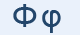Greek Letter Phi (Φι - Φ)The Ancient Greeks had a letter ("digamma", which means two gammas, one on top of the other) for the "f" sound but eventually dropped it. The Romans revived it, and therefore languages deriving from latin did not need a special letter for "ph". By custom, the Greek origin of a word is indicated by using the "ph" letter-pair. The Greeks made up this letter and the remaining three, and added them to the end of their alphabet.

"Φ" Letter in mathematics and in science

Φ represents:

• a wave function
• magnetic flux

φ represents:

• the golden ratio 1.618... in mathematics, art, and architecture
• Euler's totient function in number theory
• the argument of a complex number in mathematics
• the value of a plane angle in physics and mathematics
• the angle to the z axis in spherical coordinates
• latitude in geodesy
• a wave function
• electric potential

Greek words with "φ"

φακός, φωτιά